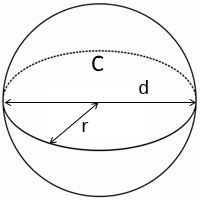# Sphere Volume and Area Calculator

Use this calculator to find out the volume and area of a sphere using either the sphere's radius, diameter or circumference.

## Sphere Volume and Area

The area `A` of a sphere is calculated using the formula:

`A = 4pir^2`, where `r` is the radius of the sphere.

The volume `V` of a sphere is calculated using the formula:

`V = (4pir^3)/3`

If the diameter of the sphere `d` and the length of the circumference `C` are known, its area can be calculated (without the figure `pi`) using the formula:`A=Cd`

and the volume using the formula:

`V = (Cd^2)/6`